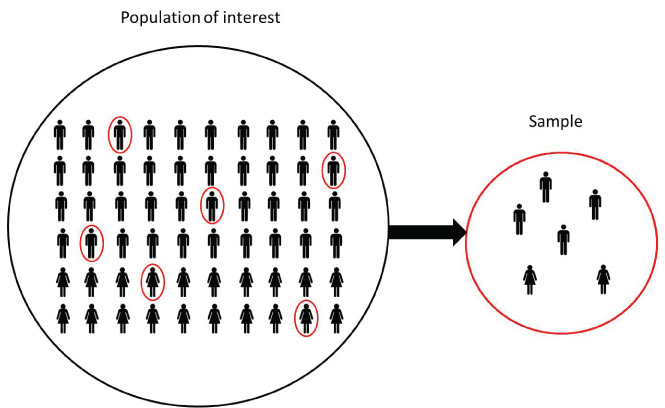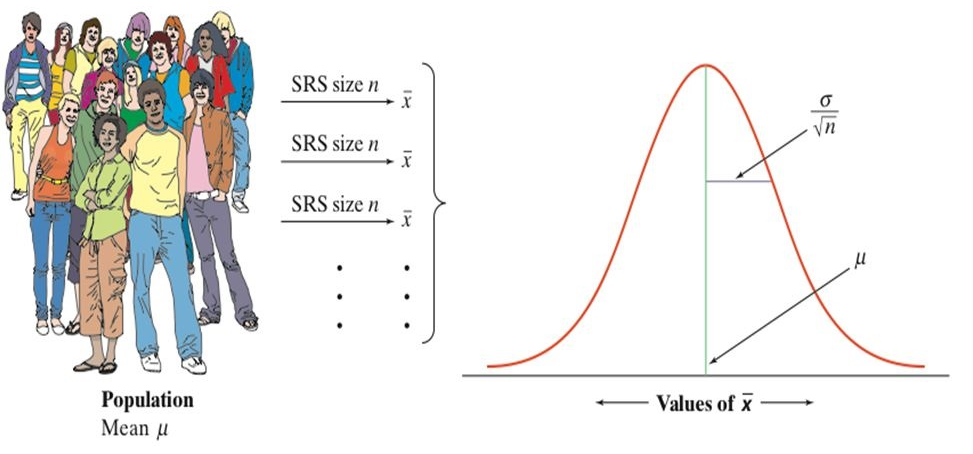# Learning objectives

• Population parameters and sample statistics
• Sampling distributions
• The Central Limit Theorem
• Exercises

## 1.1 Statistical inference

In statistical inference we are interested in learning some quantity representing some feature or parameter of a population. Population is large and we cannot examine all the values in the population directly. Therefore, to learn about the population parameter we take samples from the population and use the information in the samples to draw conclusions about the population.Population parameters are fixed values. We rarely know the parameter values because it is often difficult to obtain measures from the entire population.

Sample statistics are quantities computed from the values in the sample. They are random variables because they vary from sample to sample.

We use sample statistics to draw conclusions about unknown population parameters.

Example

Suppose we are interested in knowing the mean height of the population living in England. Population is large and we cannot measure the height of everybody directly. We can get an estimate of the mean height by taking sample of say, 5000 people, and measuring their heights.

We can provide point estimates and interval estimates for population parameters.

• Population mean is denoted by $$\mu$$ and it is unknown

• Sample size is $$n = 5000$$

• Heights of people in the sample: $$(x_1, x_2, \ldots, x_{5000})$$

• Point estimate = estimate that specifies a single value of the population.

• Sample statistic is the average heights of people in the sample: $$\bar x = \sum x_i/n$$

• Interval estimate = estimate that specifies a range of plausible values for the population parameter. We are 95% confident the population mean $$\mu$$ is within $$(a, b)$$

Example

A survey is carried out at a university to estimate the proportion of undergraduate students who drive to campus to attend classes. One thousand students are randomly selected and asked whether they drive or not to campus to attend classes. The population is all of the undergraduates at that university. The sample is the group of 1000 undergraduate students surveyed. The parameter is the true proportion of all undergraduate students at that university who drive to campus to attend classes. The statistic is the proportion of the 1000 sampled undergraduates who drive to campus to attend classes.

• Population proportion $$p$$ unknown
• Sample size $$n = 1000$$
• Sample statistic is the sample proportion: $$\hat p = \frac{\mbox{number people drive}}{n}$$

Example

A study is conducted to estimate the true mean annual income of all adult residents of California. The study randomly selects 2000 adult residents of California. The population consists of all adult residents of California. The sample is the 2000 residents in the study. The parameter is the true mean annual income of all adult residents of California. The statistic is the mean income of the 2000 residents in this sample.

• Population mean $$\mu$$ unknown
• Sample size $$n = 2000$$
• Sample statistic is the sample mean: $$\bar x = \sum x_i/n$$

## 1.2 Sampling distributions of sample statistics

A sample statistic is a random variable because it varies from sample to sample. The distribution of a sample statistic is called sampling distribution.

The sampling distribution of a sample statistic has mean approximately equal to the population mean and standard deviation called standard error (SE).

Steps to construct the sampling distribution of a sample statistic (e.g., sample mean, sample proportion, etc.):

1. Take a random sample of size $$n$$ from the population
2. For each sample, calculate the sample statistic (e.g., sample mean, sample proportion, etc.)
3. Return the sample observations to the population and repeat the process many times
4. If (theoretically) we choose every possible sample of size $$n$$ from the population and calculate the sample statistic, we obtain the sampling distribution of the sample statistic

## 1.3 The Central Limit Theorem (CLT)

The Central Limit Theorem states that for any given population distribution with mean $$\mu$$ and standard deviation $$\sigma$$, if we randomly take sufficiently large ($$n \geq 30$$) random samples from the population with replacement, the sampling distribution of the sample mean $$\bar X$$ is normally distributed with mean $$\mu$$ and standard error SE = $$\sigma/\sqrt{n}$$.

When we choose many random samples from a population, the sampling distribution of the sample mean $$\bar X$$ is centered at the population mean $$\mu$$ and is less spread out than the population distribution.

$\bar X \sim N\left(\mu, \frac{\sigma}{\sqrt{n}}\right)$Example

Consider a sample of size $$n=40$$ from a population with mean $$\mu = 30$$ and standard deviation $$\sigma=10$$. What are the mean and standard deviation of the sample mean?

Solution:

$$\mu_{\bar X} = \mu = 30$$

$$\sigma_{\bar X} = \sigma/\sqrt{n} = 10/\sqrt{40}$$

$$\bar X \sim N(\mu = 30, \sigma = 10/\sqrt{40})$$

Example

The mean height of a population is 160cm with standard deviation of 10. If we sample 50 people, what is the probability that the average height of the sample is greater than 170cm?

Solution:

$$\mu_{\bar X} = \mu = 160$$

$$\sigma_{\bar X} = \sigma/\sqrt{n} = 10/\sqrt{50}$$

$$\bar X \sim N(\mu = 160, \sigma = 10/\sqrt{50})$$

The probability that the average height of the sample is greater than 170cm is $$P(\bar X > 170)$$.

### 1.3.1 Simulation Central Limit Theorem

Consider a population with mean $$\mu = 10$$ and standard deviation $$\sigma = 3$$ (size N = 20000).Choose a large number of samples of size $$n=50$$ from the population

Sample 1

##   10.543958 12.571082 12.742054 13.848933  8.461401  8.325682  8.747708
##   13.260911  7.850794  4.971409  2.175708 11.344404  5.103495 10.443574
##  12.177068  5.944128  8.443244  8.170394 12.270357  9.563444 11.446580
##   8.575540  8.749251  3.489630 13.417128  7.769008 11.251782 14.012025
##   4.962653  8.361082  5.951567  5.611529 15.781981  8.686700  6.415523
##   9.094323  7.479232 10.005663 12.962503  9.677652  5.816455 13.830049
##   9.794368 10.296522 14.718460  9.745052  8.847465  9.350323 11.645790
##  12.262989

Sample 2

##   10.259867  7.478061 12.720841  9.046628 14.978180 10.422174  6.098966
##   12.475435  9.733125 13.013375  7.802642 11.677517  8.065506 11.815551
##  10.614832 10.630232 13.320632 11.118829  8.140150 10.263650  8.459754
##  14.286191  4.639782 11.246295 11.912051  4.252838  7.435546 10.004882
##  11.966693 11.006743 12.450126  9.361934 10.214404 11.128480  6.938571
##   9.269346 12.912279 11.240477  9.775512 10.767877 17.035887 12.725600
##   5.572606 12.521181  7.348265 10.579583  6.676816 16.887545  7.126414
##   8.936346

Sample 1000

##   13.662354  4.181706 10.552880 13.694963 14.969899 13.013250 12.245547
##    6.920323  6.614342  7.474592  9.143809 10.509498 13.598895 13.384251
##  10.102632  6.719265  7.430073 11.250937 10.122640 10.604156  8.621805
##   9.278110 14.853600 12.014415  9.461022  7.132098 13.693625  7.445386
##   4.651857  4.701105 10.698758 14.174123 10.375613 11.150260  2.853950
##  14.876582  8.386776  7.738389 12.799983 10.261418 11.899638 10.504154
##  14.946504 10.213273 10.327836  9.327777 12.625879  7.352156  8.444737
##   4.630962

Calculate sample means

$$\bar X$$ sample 1

##  9.539372

$$\bar X$$ sample 2

##  10.28712

$$\bar X$$ sample 1000

##  10.03276
• The mean of the sample means is equal to the mean of the population. $\mu_{\bar X} = \mu$ (independent of sample size)

$$\mu_{\bar X}$$

##  9.977229

$$\mu$$

##  9.981248
• The standard deviation of sample means is equal to the standard deviation of the population divided by the square root of the sample size $\sigma_{\bar X} =\frac{\sigma}{\sqrt{n}}$

$$\sigma_{\bar X}$$

##  0.4121417

$$\frac{\sigma}{\sqrt{n}}$$

##  0.4235469
• If the population is normal, then the sample means will have a normal distribution

• If the population is not normal (can be skewed, discrete, etc) but the sample is sufficiently large ($$n \geq 30$$), then the sampling distribution of sample means approximates a normal distribution.

Sampling distribution of sample means### 1.3.2 Simulation Central Limit Theorem

We illustrate the Central Limit Theorem (CLT) by means of a simulation. The CLT applies to any distribution. To illustrate the CLT we can consider any population, it does not necessarily need to be normal. For example, we consider the following population which consists of 100000 numbers drawn from two different normal distributions.

pop <- c(rnorm(20000, mean = 10, sd = 3),
rnorm(80000, mean = 70, sd = 10))

hist(pop, main = "Population")The mean and standard deviation of the population are the following:

mean(pop)
##  57.98865
sd(pop)
##  25.62395

Now we randomly select 1000 samples of size $$n=50$$.

n <- 50
mats <- NULL
for(i in 1:1000){
s <- sample(pop, n, replace = TRUE)
mats <- cbind(mats, s)
}

We calculate the mean of each sample and plot the sampling distribution of the sample mean.

samplemeans <- apply(mats, 2, mean)
hist(samplemeans, main = "Sample mean")We calculate the mean and standard deviation of the sampling distribution of the sample mean and check the CLT.

• Sample size $$n \geq 30$$.

• Sampling distribution of sample mean approximates to a normal distribution.

• Mean of the sample mean is $$\mu_{\bar X} = \mu$$

mean(samplemeans)
##  57.78448
mean(pop)
##  57.98865
• Standard error (standard deviation of the sample mean) is $$\sigma_{\bar X} =\frac{\sigma}{\sqrt{n}}$$
sd(samplemeans)
##  3.450763
sd(pop)/sqrt(n)
##  3.623774